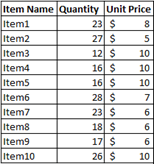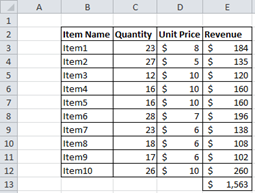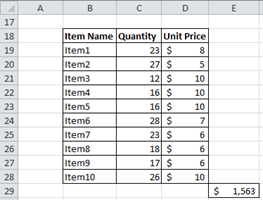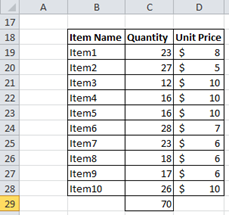# Sumproduct Function

Sum product function lets you calculate sum of values with corresponding arrays. For example, if you have sales quantites and unit prices for a series of products, you can calculate total revenue with just one formula.

Lets assume our data looks like this:If we want to calculate total revenue with traditional way, we first have to calculate revenue by item and than calculate total revenue by summing them. For this data, it takes 11 formulas to achive that.With the use of Sumproduct Function, you can do this calculation with one formula:

=SUMPRODUCT(C19:C28;D19:D28)

=SUMPRODUCT(Quantity Range;Unit Price Range)There is an important thing to remember about sumproduct function though. For sumproduct function to work, both ranges should have same lenght. If one range has 4 values and the other has 5 values, you will get a #VALUE error.

Using sumproduct with filter is also possible (like sumif function). If you’d like to sum quantites for items with 10\$ unit price, you can use the formula below.

=SUMPRODUCT((C19:C28)*(D19:D28=10))Like shown in the picture above, it will sum quantites of items with 10\$ unit price.

#### You may also like...

This site uses Akismet to reduce spam. Learn how your comment data is processed.